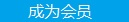您可以捐助，支持我们的公益事业。 1元 10元 50元 认证码：必填求知 文章 文库 Lib 视频 iProcess 课程 认证 咨询 工具 讲座 Modeler Code要资料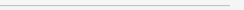订阅捐助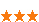732 次浏览     评价： 好 中 差
2018-8-30

 编辑推荐: 本文来自于网络，介绍了如何选择并训练模型，处理数据，以及在终端移动设备上工程化落地深度神经网络并做GPU加速，。

AI无疑是近几年的超级风口，“All in AI“等押宝AI的口号层出不穷，AI的风头一时无两。实现AI有很多种途径方法，这其中深度学习神经网络被认为是最有可能实现AI的途径。作为工程人出身的我们，更是迫切需要主动去迎接AI时代的到来，带着智能图像识别的需求，我们尝试去工程化深度神经网络并最终落地，当中的一些实践经验通过本文记录下来。

MobileNet模型

MobileNet是谷歌为移动终端设备专门设计的高效深度神经网络模型，整个模型的参数量以及运算量都控制的比较小，并且在图像分类和物体检测等任务上均有着非常不错的效果。基于MobileNet模型在移动终端设备上良好的性能，我们最终选择使用MobileNet来实现图片分类的功能（PS：我们选择的MobileNet版本是MobileNet V1，因此这里的MobileNet指的是MobileNet V1）。

MobileNet的模型结构如下图所示：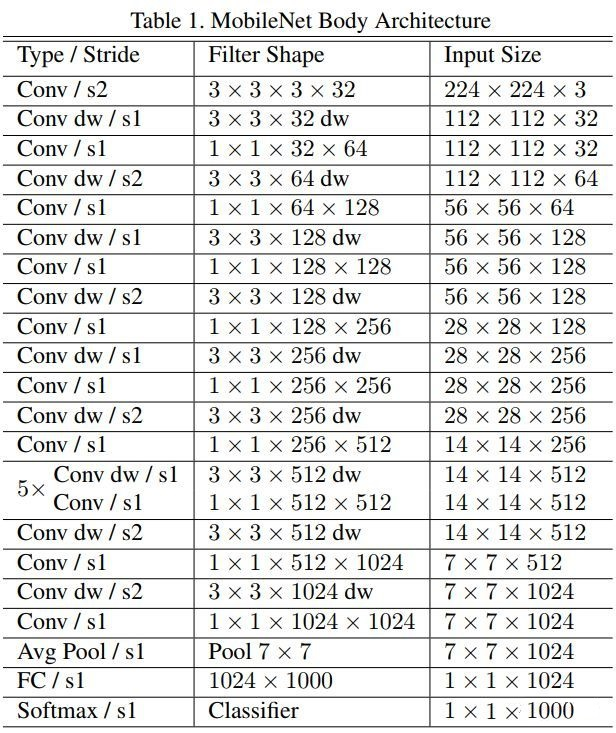MobileNet是基于深度可分离卷积的，即把一个标准的卷积拆分成一个深度卷积（depthwise convolution）和一个逐点卷积（pointwise convolution），如图：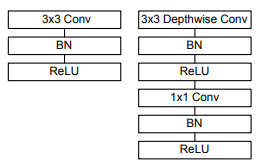MobileNet在第一层普通卷积层后，后面进行了多次深度可分离卷积，这么做的好处是在保留了图像特征的同时大幅度降低了模型的参数量和计算量。这是如何做到的呢？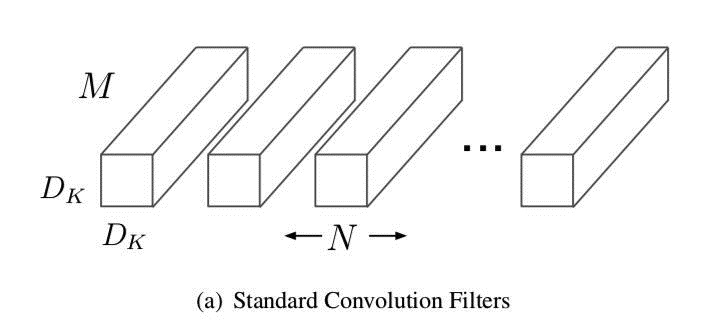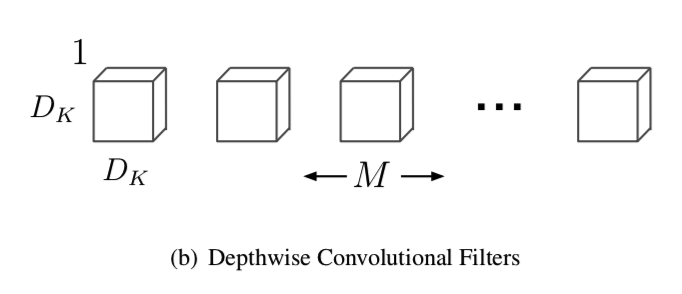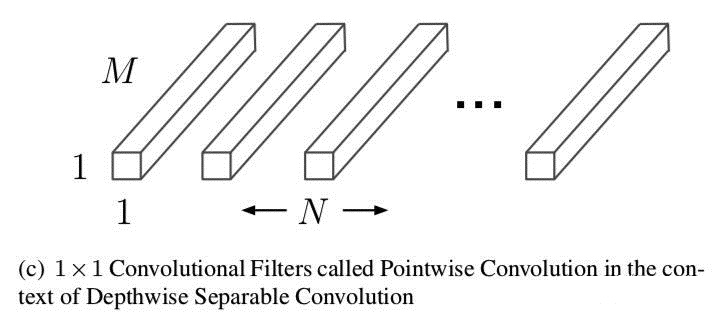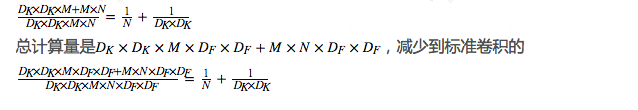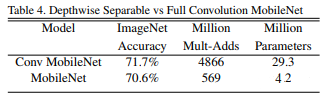int output_count = output_width * output_height * input_channel; float *output = (float *)malloc(output_count * sizeof(float)); int index = 0; for (int i = 0; i < output_height; ++i) { for (int j = 0; j < output_width; ++j) { for (int k = 0; k < input_channel; ++k) { float sum = bias[k]; for (int m = 0; m < kernel_size; ++m) { for (int n = 0; n < kernel_size; ++n) { int ypos = i * stride + m - padding; int xpos = j * stride + n - padding; if (ypos < 0 || ypos >= input_height) { continue; } if (xpos < 0 || xpos >= input_width) { continue; } float x = input[ypos * input_width * input_channel + xpos * input_channel + k]; float w = weights[(m * kernel_size + n) * input_channel + k]; sum += w * x; } } sum = fmax(sum, 0.f); sum = fmin(sum, 6.f); output[index++] = sum; } } }

MobileNet是开源

(https://github.com/tensorflow/models/blob
/master/research/slim/nets/mobilenet_v1.py)的，TensorFlow对MobileNet有非常好的支持（毕竟都是谷歌自己的东西），基于TensorFlow的slim模块代码实现非常的简洁优雅，并且还公开了预训练好的模型。但预训练好的模型是用于识别1001种类别，并不是我们自己想要的图片类别，为此，我们需要专门训练出用于识别指定图片类别的MobileNet模型。

1.开源数据集

2.数据上报

3.爬虫抓取

1.分工筛选分类

2.使用数据标注工具

1.过拟合

2.欠拟合

3.训练速度慢

4.内存不足

(https://github.com/tensorflow/hub/blob/master/
examples/image_retraining/retrain.py)。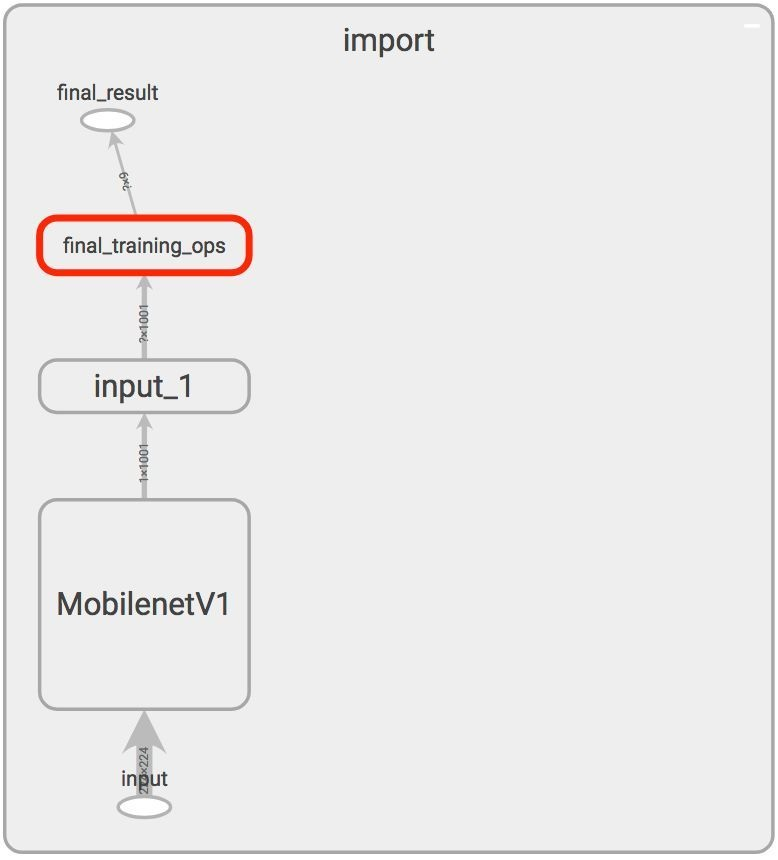Tensorflow的模型一般为pb格式，图数据和参数数据都固化在pb文件里，Tensorflow提供了命令行，可以把pb文件转化成Tensorflow Lite支持的tflite文件。要顺利运行命令行，需要先安装构建工具Bazel(https://bazel.build/)，接着执行：

 bazel run --config=opt \ //tensorflow/contrib/lite/toco:toco -- \ --input_file=/tmp/mobilenet_v1_0.50_128/frozen_graph.pb \ --output_file=/tmp/foo.tflite \ --input_format=TENSORFLOW_GRAPHDEF \ --output_format=TFLITE \ --inference_type=FLOAT \ --input_shape=1,128,128,3 \ --input_array=input \ --output_array=MobilenetV1/Predictions/Reshape_1

 bazel run --config=opt \ //tensorflow/contrib/lite/toco:toco -- \ --input_file=/tmp/some_quantized_graph.pb \ --output_file=/tmp/foo.tflite \ --input_format=TENSORFLOW_GRAPHDEF \ --output_format=TFLITE \ --inference_type=QUANTIZED_UINT8 \ --input_shape=1,128,128,3 \ --input_array=input \ --output_array=MobilenetV1/Predictions/Reshape_1 \ --mean_value=128 \ --std_value=127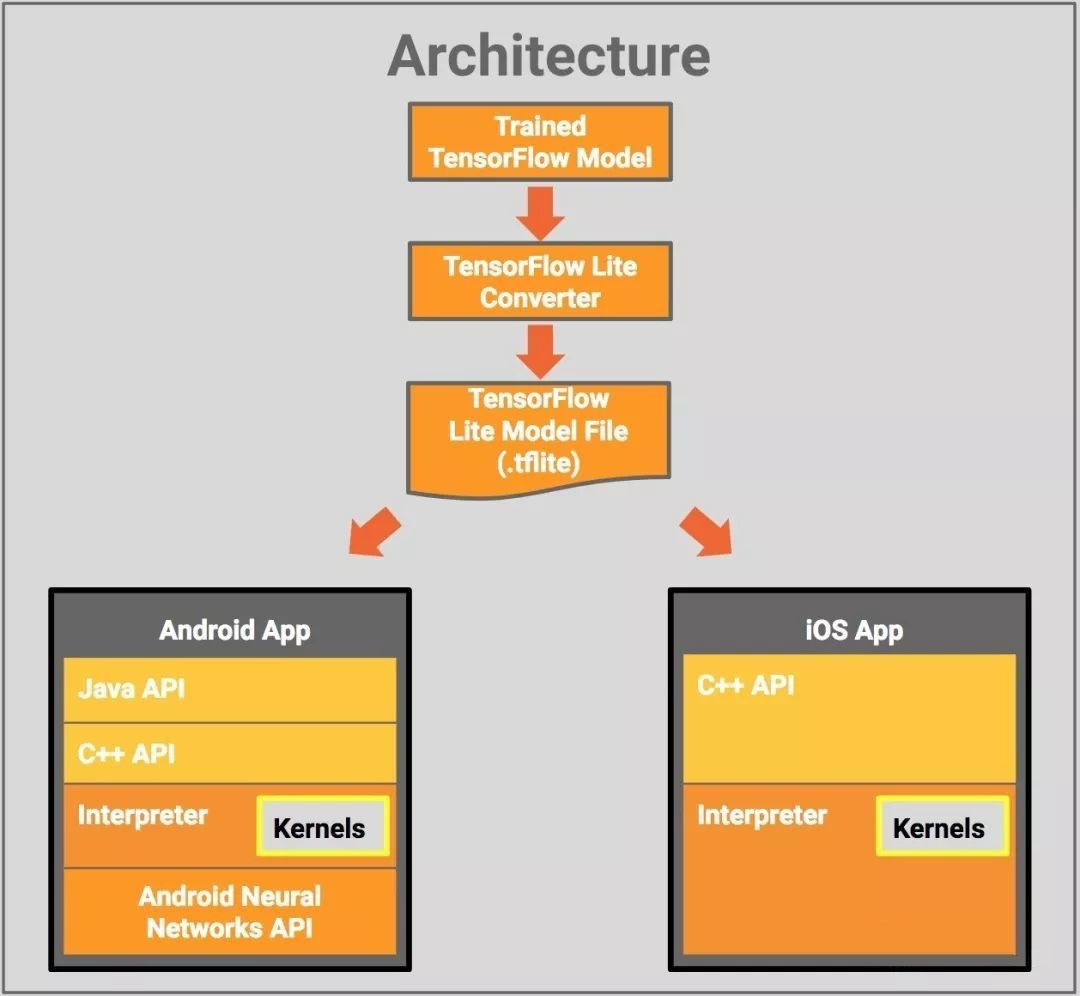Tensorflow Lite在Android上是以.so的形式集成进app，iOS上则是以静态库.a的形式集成进app。集成了Tensorflow Lite库的app就可以调用相关API来加载并运行模型。此外，官方提供了Tensorflow Lite的sample例子

(https://github.com/tensorflow/tensorflow/tree/
master/tensorflow/contrib/lite/examples)，以iOS平台为例，首先是加载模型和图像分类信息：

 NSString* graph_path = FilePathForResourceName(model_file_name, @"tflite"); model = tflite::FlatBufferModel::BuildFromFile([graph_path UTF8String]); if (!model) { LOG(FATAL) << "Failed to mmap model " << graph_path; } LOG(INFO) << "Loaded model " << graph_path; model->error_reporter(); LOG(INFO) << "resolved reporter"; tflite::ops::builtin::BuiltinOpResolver resolver; LoadLabels(labels_file_name, labels_file_type, &labels); tflite::InterpreterBuilder(*model, resolver)(&interpreter); if (!interpreter) { LOG(FATAL) << "Failed to construct interpreter"; } if (interpreter->AllocateTensors() != kTfLiteOk) { LOG(FATAL) << "Failed to allocate tensors!"; }

 int input = interpreter->inputs(); uint8_t* out = interpreter->typed_tensor(input); for (int y = 0; y < wanted_input_height; ++y) { uint8_t* out_row = out + (y * wanted_input_width * wanted_input_channels); for (int x = 0; x < wanted_input_width; ++x) { const int in_x = (y * image_width) / wanted_input_width; const int in_y = (x * image_height) / wanted_input_height; uint8_t* in_pixel = in + (in_y * image_width * image_channels) + (in_x * image_channels); uint8_t* out_pixel = out_row + (x * wanted_input_channels); for (int c = 0; c < wanted_input_channels; ++c) { out_pixel[c] = in_pixel[c]; } }

 if (interpreter->Invoke() != kTfLiteOk) { LOG(FATAL) << "Failed to invoke!"; }

 const int output_size = 1000; const int kNumResults = 5; const float kThreshold = 0.1f; std::vector> top_results; uint8_t* output = interpreter->typed_output_tensor(0); GetTopN(output, output_size, kNumResults, kThreshold, &top_results);

GPU加速模型

GPU编程技术选择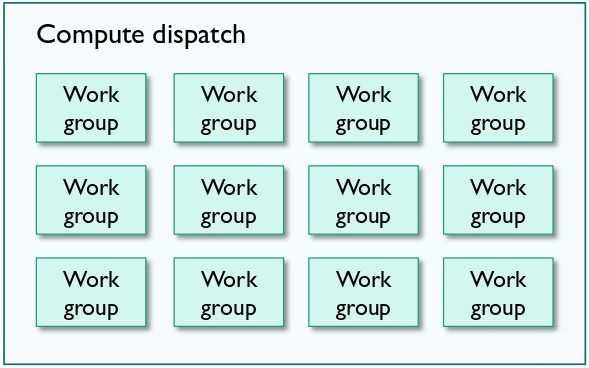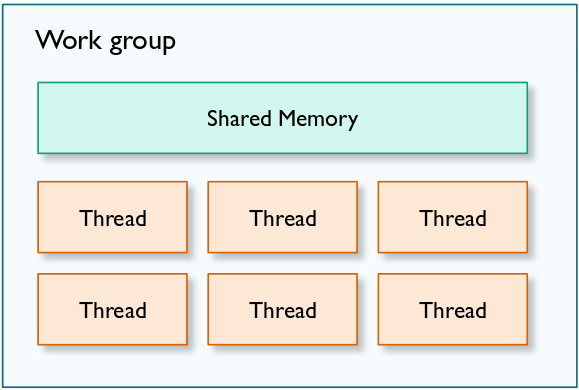kernel void depthwiseConv_quantized( texture2d_array inTexture [[texture(0)]], texture2d_array outTexture [[texture(1)]], constant KernelParams& params [[buffer(0)]], const device int* weights [[buffer(1)]], const device int4* biasTerms [[buffer(2)]], ushort3 gid [[thread_position_in_grid]]) { ushort idx = gid.x; ushort idy = gid.y; ushort idz = gid.z; if (idx >= outTexture.get_width() || idy >= outTexture.get_height() || idz >= outTexture.get_array_size()) return; const ushort kernel_size = params.kernelSize; const ushort input_width = params.inputWidth; const ushort input_height = params.inputHeight; const ushort stride = params.stride; const ushort padding = params.padding; const int inputOffset = params.inputOffset; int4 sum = int4(biasTerms[idz]); for (ushort i = 0; i < kernel_size; ++i) { for (ushort j = 0; j < kernel_size; ++j) { ushort ypos = gid.y * stride + i - padding; ushort xpos = gid.x * stride + j - padding; if (ypos < 0 || ypos >= input_height) { continue; } if (xpos < 0 || xpos >= input_width) { continue; } int4 input = int4(inTexture.read(ushort2(xpos, ypos), idz)) - inputOffset; int wx = int(weights[(idz * 4 + 0) * kernel_size * kernel_size + i * kernel_size + j]); int wy = int(weights[(idz * 4 + 1) * kernel_size * kernel_size + i * kernel_size + j]); int wz = int(weights[(idz * 4 + 2) * kernel_size * kernel_size + i * kernel_size + j]); int ww = int(weights[(idz * 4 + 3) * kernel_size * kernel_size + i * kernel_size + j]); sum.x += (input.x * wx); sum.y += (input.y * wy); sum.z += (input.z * wz); sum.w += (input.w * ww); } } sum.x = MultiplyByQuantizedMultiplierSmallerThanOne(sum.x,params.multiplier,params.shift); sum.y = MultiplyByQuantizedMultiplierSmallerThanOne(sum.y,params.multiplier,params.shift); sum.z = MultiplyByQuantizedMultiplierSmallerThanOne(sum.z,params.multiplier,params.shift); sum.w = MultiplyByQuantizedMultiplierSmallerThanOne(sum.w,params.multiplier,params.shift); sum += params.outputOffset; sum = max(0, sum); sum = min(255, sum); outTexture.write(half4(sum), gid.xy, idz); }

Android的实现代码片段如下：

 void main(){ int idx = int(gl_GlobalInvocationID.x); int idy = int(gl_GlobalInvocationID.y); int idz = int(gl_GlobalInvocationID.z); int weightPos = kernelSize * kernelSize * idz; int sum = bias.data[idz]; int tempx,tempy; for(int i = 0;i< kernelSize;i++ ) { tempx = idx*stride + i - padding; for(int j = 0; j < kernelSize; j++) { tempy = idy*stride + j - padding; if (tempx <0 || tempx >= input_height || tempy < 0 || tempy >= input_width) continue; int weight = weights.data[weightPos + i * kernelSize + j]; int inputv = input0.data[tempx * input_width * output_channel + tempy * output_channel + idz]; sum += (inputv + inputOffset) * (weight); } } sum = MultiplyByQuantizedMultiplierSmallerThanOne(sum,multiplier,shift); sum += outputOffset; sum = max(0, sum); sum = min(255, sum); output0.data[idz + idy * output_channel + idx * output_width * output_channel] = sum; }

GPU加速效果732 次浏览  评价: 好 中 差订阅捐助
 相关文章 我们该如何设计数据库 数据库设计经验谈 数据库设计过程 数据库编程总结
 相关文档 数据库性能调优技巧 数据库性能调整 数据库性能优化讲座 数据库系统性能调优系列
 相关课程 高性能数据库设计与优化 高级数据库架构师 数据仓库和数据挖掘技术 Hadoop原理、部署与性能调优每天2个文档/视频 扫描微信二维码订阅
 订阅技术月刊 获得每月300个技术资源